#### Option 3.2.8. Equation w/Override

Select Equation w/Override in the Calculation field on the Virtual tab of the Point Properties dialog box.

An Equation w/Override point is similar to an Equation point. It uses the expression you specify in the Expression field to update the point's value.

In addition, applications may change the value of an Equation w/Override point. The changed value remains in effect until one of the source points in the Expression changes and the Expression is recalculated, or until an application supplies a new value for the point.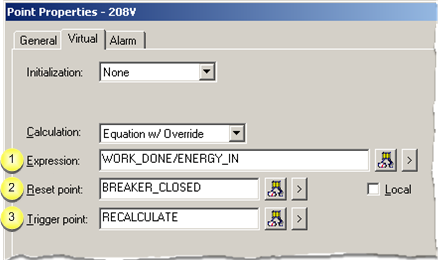Expression Reset point Trigger point

 Expression

Enter an expression that calculates the value of the Equation w/Override point.

The expression will be monitored for transitions.

The expression may contain: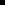One or more Point IDs along withConstant values, operations, and functions.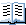Rules for defining an expression are:

1. Avoid introducing loops (circular references between points) in your expressions.

Example of what to AVOID:

VIRTUAL_PT1 = DEVICE_PT + VIRTUAL_PT2

VIRTUAL_PT2 = DEVICE_PT + VIRTUAL_PT1

Although this will not cause CIMPLICITY software to fail, it will cause the Virtual Point Processor to always have work to do. This causes all available computer time to be consumed, which adversely impacts system performance.

1. Use proper formatting for array points.

Example of the correct format

VIRTUAL_PT1 = DEVICE_PT / 2.0

1. Use proper formatting for Point IDs with reserved words.

Point IDs that are reserved words, or contain spaces, arithmetic operators, parentheses, or that begin with a non-alphabetic character must be placed in single quotes when used in an expression.

Example

VIRTUAL_PT2 = 'DEVICE_PT:0' * 'WARNING_LOW'

Buttons to help with the equation are as follows.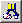Opens the Expression Editor. Displays a Popup menu to: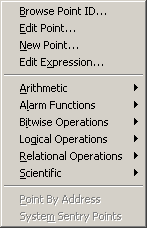Open: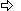The Select a Point browser.The Point Properties dialog box for a selected point.A New Point dialog box.The Expression Editor.Display an extended menu with the associated operations.

 Reset point

Based on whether there is an entry in the Reset Point field, at runtime the Equation /Override point is reset as follows:

 When the trigger point is not defined If And Then An Initial Equation w/Override Point Value is The Current value of the expression is The Equation w/Override point value is reset to the: Not defined Unavailable Zero Not defined Available Current value Defined Unavailable Current value Defined Available Current value When the trigger point is defined If Then An Initial Equation w/Override Point Value is The Equation w/Override point value is reset to the: Not defined Zero Defined Initial value

Note: Buttons to the right of the Reset Point and Trigger Point fields help with configuration.Opens the Select a Point browser to select an available point. Displays a Popup menu to: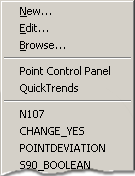Open:A New Point dialog box.The Point Properties dialog box for a selected point.The Select a Point browser.Select a recently selected point.

 Trigger point

When the value of the Equation w/Override updates depends on whether or not you defined the trigger point as follows:

 Trigger point The Value of the Equation w/Override is Udated When the Value of the: Defined Trigger point is updated. Not defined Value of the source point in the Expression field is updated.

Buttons to help with the trigger point have the same functionality as the buttons to the right of the Reset Point field.

 Step 3.2. Configure virtual calculations.